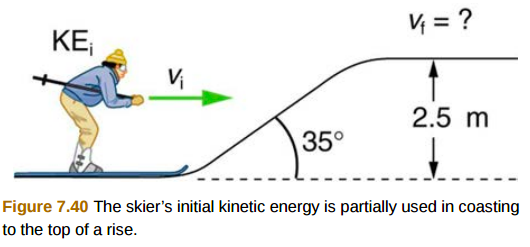# Problem: A 60.0 kg skier with an initial speed of 12.0 m/s coasts up a 2.50-m-high rise as shown in Figure 7.40. Find her final speed at the top, given that the coefficient of friction between her skis and the snow is 0.0800. (Hint: Find the distance traveled up the incline assuming a straight-line path as shown in the figure.)

###### FREE Expert Solution

In this problem, we are moving up an incline against both conservative and non-conservative forces.

We'll need the conservation of energy equation.

Law of conservation of energy:

$\overline{){{\mathbf{K}}}_{{\mathbf{i}}}{\mathbf{+}}{{\mathbf{U}}}_{{\mathbf{i}}}{\mathbf{+}}{{\mathbf{W}}}_{{\mathbf{nc}}}{\mathbf{=}}{{\mathbf{K}}}_{{\mathbf{f}}}{\mathbf{+}}{{\mathbf{U}}}_{{\mathbf{f}}}}$, where Wnc is the work done by non-conservative forces such as friction.

Work done:

$\overline{){\mathbf{W}}{\mathbf{=}}{\mathbf{f}}{\mathbf{d}}}$

94% (246 ratings)###### Problem Details

A 60.0 kg skier with an initial speed of 12.0 m/s coasts up a 2.50-m-high rise as shown in Figure 7.40. Find her final speed at the top, given that the coefficient of friction between her skis and the snow is 0.0800. (Hint: Find the distance traveled up the incline assuming a straight-line path as shown in the figure.)